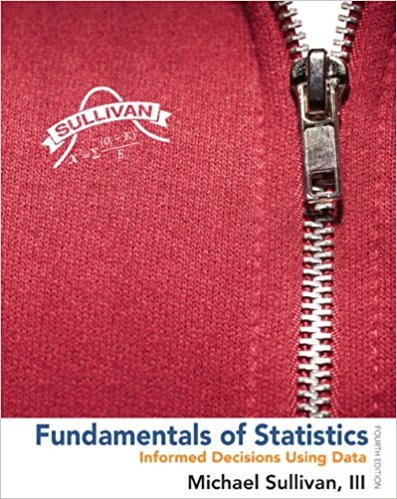×
×

# Solutions for Chapter Chapter 10.4: Fundamentals of Statistics 4th Edition## Full solutions for Fundamentals of Statistics | 4th Edition

ISBN: 9780321838704Solutions for Chapter Chapter 10.4

Solutions for Chapter Chapter 10.4
4 5 0 261 Reviews
18
4
##### ISBN: 9780321838704

Fundamentals of Statistics was written by and is associated to the ISBN: 9780321838704. This expansive textbook survival guide covers the following chapters and their solutions. This textbook survival guide was created for the textbook: Fundamentals of Statistics, edition: 4. Since 46 problems in chapter Chapter 10.4 have been answered, more than 268345 students have viewed full step-by-step solutions from this chapter. Chapter Chapter 10.4 includes 46 full step-by-step solutions.

Key Statistics Terms and definitions covered in this textbook
• Acceptance region

In hypothesis testing, a region in the sample space of the test statistic such that if the test statistic falls within it, the null hypothesis cannot be rejected. This terminology is used because rejection of H0 is always a strong conclusion and acceptance of H0 is generally a weak conclusion

• Alternative hypothesis

In statistical hypothesis testing, this is a hypothesis other than the one that is being tested. The alternative hypothesis contains feasible conditions, whereas the null hypothesis speciies conditions that are under test

• Arithmetic mean

The arithmetic mean of a set of numbers x1 , x2 ,…, xn is their sum divided by the number of observations, or ( / )1 1 n xi t n ? = . The arithmetic mean is usually denoted by x , and is often called the average

• Binomial random variable

A discrete random variable that equals the number of successes in a ixed number of Bernoulli trials.

• Cause-and-effect diagram

A chart used to organize the various potential causes of a problem. Also called a ishbone diagram.

• Coeficient of determination

See R 2 .

• Comparative experiment

An experiment in which the treatments (experimental conditions) that are to be studied are included in the experiment. The data from the experiment are used to evaluate the treatments.

• Conidence level

Another term for the conidence coeficient.

• Consistent estimator

An estimator that converges in probability to the true value of the estimated parameter as the sample size increases.

• Continuous random variable.

A random variable with an interval (either inite or ininite) of real numbers for its range.

• Continuous uniform random variable

A continuous random variable with range of a inite interval and a constant probability density function.

• Cumulative sum control chart (CUSUM)

A control chart in which the point plotted at time t is the sum of the measured deviations from target for all statistics up to time t

• Density function

Another name for a probability density function

• Dependent variable

The response variable in regression or a designed experiment.

• Designed experiment

An experiment in which the tests are planned in advance and the plans usually incorporate statistical models. See Experiment

• Eficiency

A concept in parameter estimation that uses the variances of different estimators; essentially, an estimator is more eficient than another estimator if it has smaller variance. When estimators are biased, the concept requires modiication.

• Expected value

The expected value of a random variable X is its long-term average or mean value. In the continuous case, the expected value of X is E X xf x dx ( ) = ?? ( ) ? ? where f ( ) x is the density function of the random variable X.

• Fisher’s least signiicant difference (LSD) method

A series of pair-wise hypothesis tests of treatment means in an experiment to determine which means differ.

• Fractional factorial experiment

A type of factorial experiment in which not all possible treatment combinations are run. This is usually done to reduce the size of an experiment with several factors.

• Geometric mean.

The geometric mean of a set of n positive data values is the nth root of the product of the data values; that is, g x i n i n = ( ) = / w 1 1 .

×